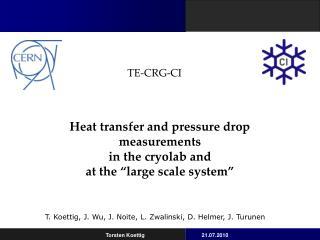# Contents - PowerPoint PPT PresentationDownload PresentationContents

ContentsDownload Presentation## Contents

- - - - - - - - - - - - - - - - - - - - - - - - - - - E N D - - - - - - - - - - - - - - - - - - - - - - - - - - -
##### Presentation Transcript

1. Contents • Progress at the large scale CO2 system, • Results of the small scale CO2 system in the cryolab.

2. CO2 - Large scale system Joao Noite, Lukasz Zwalinski, Torsten Koettig

3. CO2 - Large scale system

4. CO2 - Large scale system

5. CO2 - Large scale system • Location in 158: • - Commissioning of the system at 25°C, • Possible mass flow rate 1...15 g/s, • Run only at ambient temperature • at the moment, • Next step => accumulator to vary Tsat. Joao Noite, Lukasz Zwalinski, Torsten Koettig

6. CO2 – Small scale system Small scale system in the cryolab CERN: Test section length: 300 mm, heated part: 150 mm • Measurement of the heat transfer coefficient and pressure drop under variation of the following dependencies: • Vapor quality x • Heat flux q • Mass flux G • Saturation temperature Tsat Jihao Wu, Daniel Helmer

7. CO2 - Small scale system Heat transfer coefficient in the flow map (Tsat=263 K, G=300 kg/m2s, q=15kW/m2)

8. CO2 - Scanning the two-phase region Pressure drop in the flow map (Tsat=263 K, G=300 kg/m2s, q=15kW/m2) Pressure drop [mbar] Mass flux [kg/m2s] Vapor quality x

9. CO2 - Scanning the two-phase region • Measurement of the heat transfer coefficient and pressure drop under variation of the following dependencies: • Vapor quality x • Heat flux q • Mass flux G • Saturation temperature Tsat

10. CO2 - Scanning the two-phase region Heat flux dependency of the heat coefficient (T=263 K, G=400 kg/m2s)

11. CO2 - Scanning the two-phase region Heat flux dependency of the pressure drop(T=263 K, G=400 kg/m2s)

12. CO2 - Scanning the two-phase region • Measurement of the heat transfer coefficient and pressure drop under variation of the following dependencies: • Vapor quality x • Heat flux q • Mass flux G • Saturation temperature Tsat

13. CO2 - Scanning the two-phase region Mass flux dependency of the heat coefficient (T=263 K, q=7.5 kW/m2)

14. CO2 - Scanning the two-phase region Mass flux dependency of the pressure drop (T=263 K, q=7.5 kW/m2)

15. CO2 - Scanning the two-phase region • Measurement of the heat transfer coefficient and pressure drop under variation of the following dependencies: • Vapor quality x • Heat flux q • Mass flux G • Saturation temperature Tsat

16. CO2 - Scanning the two-phase region Temperature dependency of the heat coefficient (q=15 kW/m2, G=300 kg/m2s)

17. CO2 - Scanning the two-phase region Temperature dependency of pressure drop (q=15 kW/m2, G=300 kg/m2s)

18. Resume • Reliable test setup to determine heat transfer and pressure drop, • Measurements in the whole two phase region are done, • Influences of mass flux, heat flux and saturation temperature. Paper will be submitted to Int. Journal of Heat and Mass Transfer

19. CO2 - Small scale system Instabilities Observed instabilities: J-T expansion into subcooled liquid or x<3% oscillations occur Oscillations are amplified reaching the dryout at around 75 % vapor quality Temperatures below -34 °C